# 3.2 Domain and range  (Page 5/11)

 Page 5 / 11

Can a function’s domain and range be the same?

Yes. For example, the domain and range of the cube root function are both the set of all real numbers.

## Finding domains and ranges of the toolkit functions

We will now return to our set of toolkit functions to determine the domain and range of each.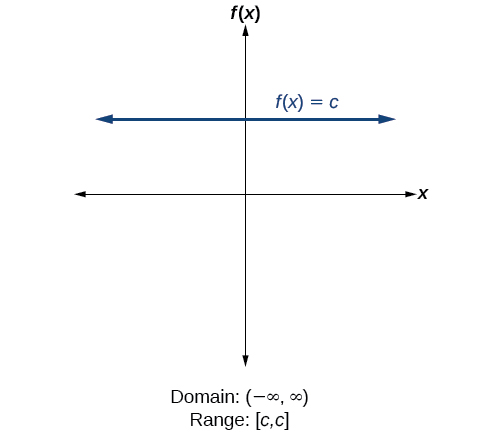For the constant function   f ( x ) = c ,   the domain consists of all real numbers; there are no restrictions on the input. The only output value is the constant   c ,   so the range is the set   { c }   that contains this single element. In interval notation, this is written as   [ c , c ] ,   the interval that both begins and ends with   c .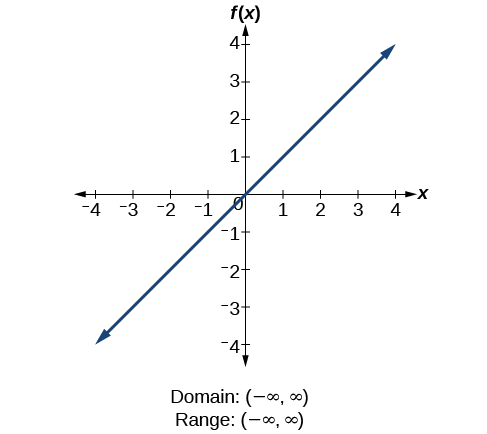For the identity function   f ( x ) = x ,   there is no restriction on   x .   Both the domain and range are the set of all real numbers.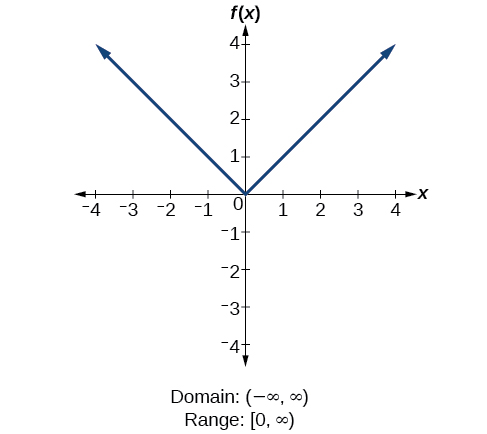For the absolute value function   f ( x ) = | x | ,   there is no restriction on   x .   However, because absolute value is defined as a distance from 0, the output can only be greater than or equal to 0.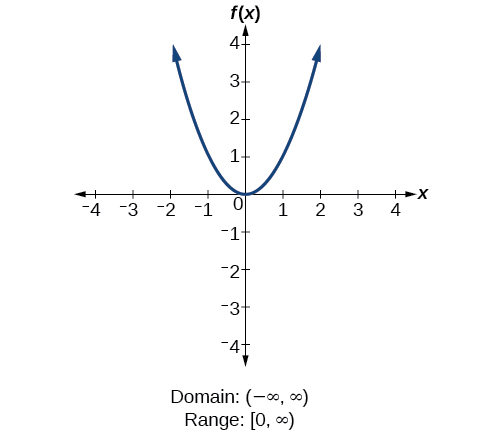For the quadratic function   f ( x ) = x 2 ,   the domain is all real numbers since the horizontal extent of the graph is the whole real number line. Because the graph does not include any negative values for the range, the range is only nonnegative real numbers.For the cubic function   f ( x ) = x 3 ,   the domain is all real numbers because the horizontal extent of the graph is the whole real number line. The same applies to the vertical extent of the graph, so the domain and range include all real numbers.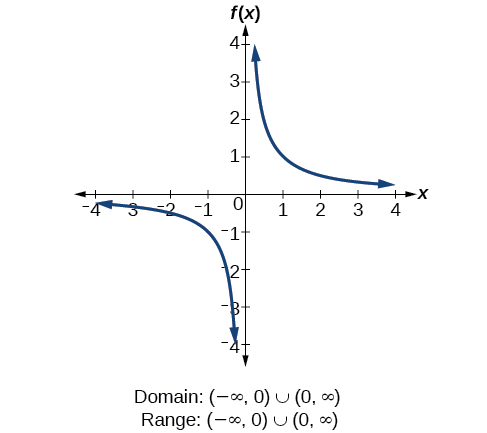For the reciprocal function   f ( x ) = 1 x ,   we cannot divide by 0, so we must exclude 0 from the domain. Further, 1 divided by any value can never be 0, so the range also will not include 0. In set-builder notation, we could also write { x |   x ≠ 0 } , the set of all real numbers that are not zero.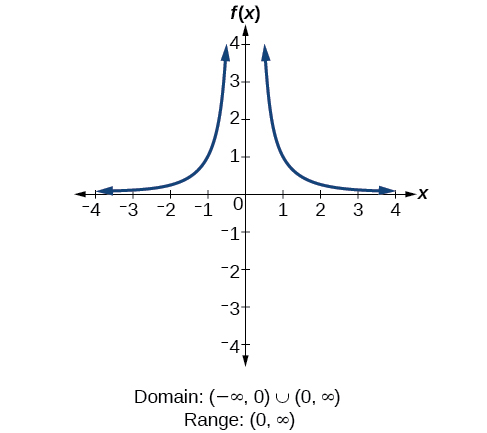For the reciprocal squared function   f ( x ) = 1 x 2 , we cannot divide by 0 , so we must exclude 0 from the domain. There is also no x that can give an output of 0, so 0 is excluded from the range as well. Note that the output of this function is always positive due to the square in the denominator, so the range includes only positive numbers.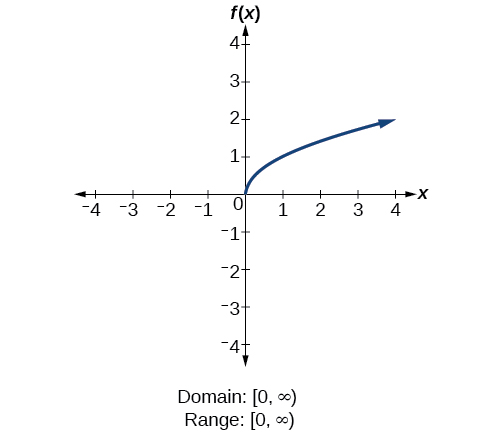For the square root function   f ( x ) = x ,   we cannot take the square root of a negative real number, so the domain must be 0 or greater. The range also excludes negative numbers because the square root of a positive number   x   is defined to be positive, even though the square of the negative number   − x   also gives us   x .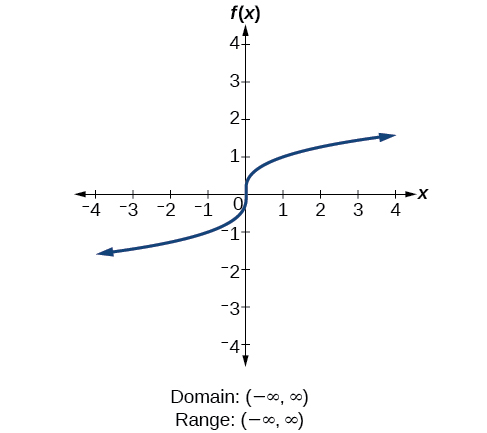For the cube root function   f ( x ) = x 3 ,   the domain and range include all real numbers. Note that there is no problem taking a cube root, or any odd-integer root, of a negative number, and the resulting output is negative (it is an odd function).

Given the formula for a function, determine the domain and range.

1. Exclude from the domain any input values that result in division by zero.
2. Exclude from the domain any input values that have nonreal (or undefined) number outputs.
3. Use the valid input values to determine the range of the output values.
4. Look at the function graph and table values to confirm the actual function behavior.

#### Questions & Answers

The sequence is {1,-1,1-1.....} has
amit Reply
circular region of radious
Kainat Reply
how can we solve this problem
Joel Reply
Sin(A+B) = sinBcosA+cosBsinA
Eseka Reply
Prove it
Eseka
Please prove it
Eseka
hi
Joel
June needs 45 gallons of punch. 2 different coolers. Bigger cooler is 5 times as large as smaller cooler. How many gallons in each cooler?
Arleathia Reply
find the sum of 28th term of the AP 3+10+17+---------
Prince Reply
I think you should say "28 terms" instead of "28th term"
Vedant
if sequence sn is a such that sn>0 for all n and lim sn=0than prove that lim (s1 s2............ sn) ke hole power n =n
SANDESH Reply
write down the polynomial function with root 1/3,2,-3 with solution
Gift Reply
if A and B are subspaces of V prove that (A+B)/B=A/(A-B)
Pream Reply
write down the value of each of the following in surd form a)cos(-65°) b)sin(-180°)c)tan(225°)d)tan(135°)
Oroke Reply
Prove that (sinA/1-cosA - 1-cosA/sinA) (cosA/1-sinA - 1-sinA/cosA) = 4
kiruba Reply
what is the answer to dividing negative index
Morosi Reply
In a triangle ABC prove that. (b+c)cosA+(c+a)cosB+(a+b)cisC=a+b+c.
Shivam Reply
give me the waec 2019 questions
Aaron Reply
the polar co-ordinate of the point (-1, -1)
Sumit Reply

### Read also:

#### Get the best Algebra and trigonometry course in your pocket!

Source:  OpenStax, Algebra and trigonometry. OpenStax CNX. Nov 14, 2016 Download for free at https://legacy.cnx.org/content/col11758/1.6
Google Play and the Google Play logo are trademarks of Google Inc.

Notification Switch

Would you like to follow the 'Algebra and trigonometry' conversation and receive update notifications?ByByByByBy